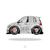# Create polygons from csv file: RuntimeError: Object: CreateObject cannot create geometry from inputs

1485
6
04-29-2022 05:24 PMNew Contributor II

So I am kind of new to python world and I'm trying to create a polygon feature class including multiple polygons from a csv file attached (parcel_points.csv) containing 3 columns: "PARCELID", "POINT_X", "POINT_Y".

I followed the code sample from https://pro.arcgis.com/en/pro-app/latest/arcpy/get-started/writing-geometries.htm. I'm currently stuck on separating each polygon by "PARCELID" but I keep getting "RuntimeError: Object: CreateObject cannot create geometry from inputs". Where did I do wrong?

``````import os
import arcpy
import fileinput

in_dir = "D:/WPS/JHU/Programming in GIS AS.430.606.81.SP22/Week 13/Final"
csvFile = "parcel_points.csv"
fc = "polygonFromCSV"
geoType = "Polygon"
in_csv = os.path.join(in_dir, csvFile)
arcpy.env.workspace = in_dir

sr = arcpy.SpatialReference(26910)
arcpy.management.CreateFeatureclass(in_dir, fc, geoType, "", "", "", sr)

with arcpy.da.InsertCursor(fc, ["SHAPE@"]) as cursor:
array = arcpy.Array()
point = arcpy.Point()

tempID = -1
for line in fileinput.input(in_csv):
if not fileinput.isfirstline():
point.ID, point.X, point.Y = line.split(",")
if tempID == -1:
tempID = line
if tempID != line:
cursor.insertRow([arcpy.Polygon(array)])
array.removeAll()
point.removeAll()
tempID = line
cursor.insertRow([arcpy.Polygon(array)])
fileinput.close()``````

Tags (2)
1 Solution

Accepted SolutionsNew Contributor III

To fix your problem you will need to alter your code to:

• Add a field to the  output feature class to store the parcel id.
• Modify the insert cursor to also update this parcel update
• Insert the row with the geometry and the parcel id every time the parcel id changes.

The  code will look like this:

``````import csv
import os
import arcpy

in_dir = "D:/demo"
csvFile = "parcel_points.csv"
fc = "polygonFromCSV"
in_csv = os.path.join(in_dir, csvFile)
sr = arcpy.SpatialReference(26910)

# Create output feature class
arcpy.management.CreateFeatureclass(
in_dir, fc, geometry_type="POLYGON", spatial_reference=sr
)
out_feature_class = os.path.join(in_dir, fc)
# Add a field to transfer PARCELID from input csv

with arcpy.da.InsertCursor(out_feature_class, ["SHAPE@", "PARCELID"]) as cursor:
point_list = []
parcel_value = None
is_first_pass = True

with open(in_csv, mode="r") as csv_file:
#print(f"Parcel id:{row} with X {row} and Y {row}.")

if is_first_pass:
parcel_value = row
is_first_pass = False
elif row != parcel_value:
# Parcel ID has changed, write the polygon
polygon = arcpy.Polygon(arcpy.Array(point_list), sr)
cursor.insertRow([polygon, parcel_value])
# Reset the current group
parcel_value = row
point_list = []

# Add the point to the feature's array of points
point_list.append(arcpy.Point(row, row))

# final insert
polygon = arcpy.Polygon(arcpy.Array(point_list), sr)
cursor.insertRow([polygon, parcel_value])``````
Mark.
6 RepliesbyMVP Esteemed Contributor
``````import numpy as np
import arcpy
name = "c:/temp/parcel_points.csv"
dt = np.dtype([('PARCELID', 'i8'), ('POINT_X', 'f8'), ('POINT_Y', 'f8')])
a = np.genfromtxt(
name, dtype=dt, delimiter=",", names=True, autostrip=True,
encoding=None)
ids = a['PARCELID']
w = np.nonzero((ids[1:] - ids[:-1] != 0))
p_lst = np.array_split(a, w)
frst = p_lst
xs = frst[['POINT_X', 'POINT_Y']].tolist()
p = arcpy.Polygon(arcpy.Array([arcpy.Point(i, i) for i in xs]))``````

'p' is the first polygon (line 11 and 12) in the 'p_list' which was obtained from splitting the array based on where the PARCELID s breakpoints occurred.

You can cobble the rest of the polygons by creating a list to hold the polygons and cycle through lines 11 to 13.... sort of retired...New Contributor II

Thank you so much for the help! I forgot to mention that the task has to be completed via insertcursor method. I believe I'm almost there since I was able to create an unseparated polygon through the code below. I'm just not able to separate them through ID.

``````import os
import arcpy
import fileinput

in_dir = "D:/demo"
csvFile = "parcel_points.csv"
fc = "polygonFromCSV"
geoType = "Polygon"
in_csv = os.path.join(in_dir, csvFile)
arcpy.env.workspace = in_dir

sr = arcpy.SpatialReference(26910)
arcpy.management.CreateFeatureclass(in_dir, fc, geoType, "", "", "", sr)

with arcpy.da.InsertCursor(fc, ["SHAPE@"]) as cursor:
array = arcpy.Array()
point = arcpy.Point()

for line in fileinput.input(in_csv):
if not fileinput.isfirstline():
point.ID, point.X, point.Y = line.split(",")
cursor.insertRow([arcpy.Polygon(array)])
fileinput.close()``````byMVP Esteemed Contributor

Has to be done by an insertcursor?

cobble the parts from above that does the splitting, then throw it into your cursor if you must

... sort of retired...New Contributor II

I have updated the code above and solved the runtime error. It was caused by false indentation. But the code still not able to separate polygons by ID. Is there anyway this code can be improved instead of rewriting the whole thing to make it work?New Contributor III

To fix your problem you will need to alter your code to:

• Add a field to the  output feature class to store the parcel id.
• Modify the insert cursor to also update this parcel update
• Insert the row with the geometry and the parcel id every time the parcel id changes.

The  code will look like this:

``````import csv
import os
import arcpy

in_dir = "D:/demo"
csvFile = "parcel_points.csv"
fc = "polygonFromCSV"
in_csv = os.path.join(in_dir, csvFile)
sr = arcpy.SpatialReference(26910)

# Create output feature class
arcpy.management.CreateFeatureclass(
in_dir, fc, geometry_type="POLYGON", spatial_reference=sr
)
out_feature_class = os.path.join(in_dir, fc)
# Add a field to transfer PARCELID from input csv

with arcpy.da.InsertCursor(out_feature_class, ["SHAPE@", "PARCELID"]) as cursor:
point_list = []
parcel_value = None
is_first_pass = True

with open(in_csv, mode="r") as csv_file:
#print(f"Parcel id:{row} with X {row} and Y {row}.")

if is_first_pass:
parcel_value = row
is_first_pass = False
elif row != parcel_value:
# Parcel ID has changed, write the polygon
polygon = arcpy.Polygon(arcpy.Array(point_list), sr)
cursor.insertRow([polygon, parcel_value])
# Reset the current group
parcel_value = row
point_list = []

# Add the point to the feature's array of points
point_list.append(arcpy.Point(row, row))

# final insert
polygon = arcpy.Polygon(arcpy.Array(point_list), sr)
cursor.insertRow([polygon, parcel_value])``````
Mark.New Contributor II

Thank you!